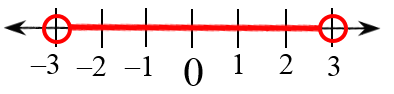### Home > A2C > Chapter 7 > Lesson 7.2.1 > Problem7-100

7-100.

Solve each inequality and graph its solution on a number line.

See the Math Notes box in Lesson 4.2.2.

1. $|x|<3$

$−31. $|2x+1|<3$

1. $|2x+1|\ge3$